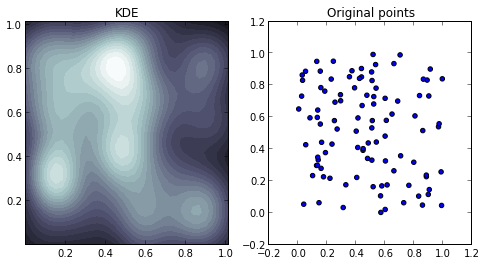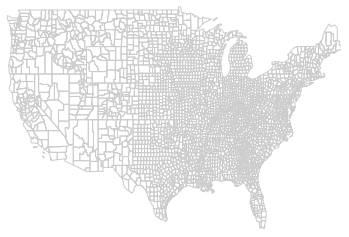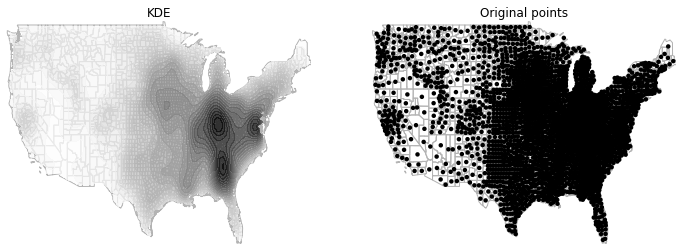# KDE for spatial data¶

This notebook presents a wrapper funtion to easily create kernel density maps from XY locations. As an additional feature, the surface obtained can be embedded into the boundaries of a .shp file.

The heavy-lifting of the kernel density estimation (KDE) is carried out by the estimator implemented in sklearn, and it leverages spatial functionality in PySAL for the boundary clipping. For an in-depth comparison of KDE implementations in Python as well as more intuition of the methodology behind it, please refer to this post by Jake VanderPlas, which was a great source of information and inspiration for this notebook.

The main functionality is implemented in a separate file named kde_maps.py. Both this notebook and the file with the code are posted as a Gist on this URL. The notebook is available under Creative Commons (see at the bottom) and the code is released under a BSD-like license (see the file).

You will need the following dependencies to use the functionality in kde_maps.py and to run this notebook:

For the notebook, all we need to import is the main method in kde_maps.py, which we assume is on the same folder as this file, pyplot, numpy and the mapping functionality in PySAL:

In :
from kde_maps import kde_grid
import numpy as np
import matplotlib.pyplot as plt
import pysal as ps
from pysal.contrib.viz import mapping as maps


## Maps without boundaries¶

The following is an example with random data that creates a kernel surface without any boundary constraint and plots it.

We first create the original points on which we are going to calculate the kernel (pts) and then estimate the surface:

In :
pts = np.random.random((100, 2))
xyz, gdim = kde_grid(pts, spaced=0.03)

x = xyz[:, 0].reshape(gdim)
y = xyz[:, 1].reshape(gdim)
z = xyz[:, 2].reshape(gdim)

levels = np.linspace(0, z.max(), 25)

Finding optimal bandwith (3-fold cross-validation):  0.15 secs
Creating grid: 0.00 secs
Evaluating kernel in grid points: 0.01 secs


Now we can display the surface as a filled contour and compare it with the original points:

In :
f = plt.figure(figsize=(8, 4))

ax1.contourf(x, y, z, levels=levels, cmap=plt.cm.bone)
ax1.set_title("KDE")

ax2.scatter(pts[:, 0], pts[:, 1])
ax2.set_title("Original points")

plt.show()## Maps within boundaries¶

In this example, we are going to visualize the density of counties in the US. For that, we will use the boundary file available in the examples folder of PySAL (NAT.shp). We extract the centroids from the polygons and create a kernel density based on them. Note that, being more than 3,000 points, clipping the grid of the surface and displaying the polygons may take a few seconds.

Before getting into the estimation, let us quickly visualize the geography we are considering:

In :
link = ps.examples.get_path("NAT.shp")Similarly as before, we can now compute the kernel:

In :
cents = np.array([poly.centroid for poly in ps.open(link)])

x = xyz[:, 0].reshape(gdim)
y = xyz[:, 1].reshape(gdim)
z = xyz[:, 2].reshape(gdim)

levels = np.linspace(0, z.max(), 25)

Finding optimal bandwith (3-fold cross-validation):  7.64 secs
2.89226078987  secs to build rtree
23.6726560593  secs to get correspondences
0.000591993331909  secs to convert correspondences
Creating grid: 26.59 secs
Evaluating kernel in grid points: 1.17 secs


And plot the surface next to the original one:

In :
shp = ps.open(link)

f = plt.figure(figsize=(12, 4))

base = maps.map_poly_shp(shp)
base.set_facecolor('none')
base.set_edgecolor('0.7')
ax1 = maps.setup_ax([base], ax=ax1)
ax1.contourf(x, y, z, levels=levels, cmap=plt.cm.Greys, alpha=0.75)
ax1.set_title("KDE")

base = maps.map_poly_shp(shp)
base.set_facecolor('none')
base.set_edgecolor('0.7')
ax2 = maps.setup_ax([base], ax=ax2)
ax2.scatter(cents[:, 0], cents[:, 1], linewidth=0, c='k')
ax2.set_title("Original points")

plt.show()Note how trying to plot the points in this case is not very useful as one quickly gets a big black blob in the NE part of the country. In this context, the use of a kernel proves very useful.

In :
from IPython.display import HTML